Search IntMath
Close

450+ Math Lessons written by Math Professors and Teachers

5 Million+ Students Helped Each Year

1200+ Articles Written by Math Educators and Enthusiasts

Simplifying and Teaching Math for Over 23 Years

# How to find the length of a spherical spiral

By Murray Bourne, 13 May 2015

I received an interesting request from reader Anne recently, involving a sphere for a Zen-style garden. She wrote: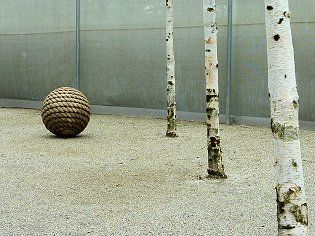I'm struggling with an applied math problem. I am going to cover the surface of a solid sphere using 1.5 cm hemp rope applied/glued in a coil such that there are no gaps. (See above photo.)

The sphere radius (uncovered) is 7.08 cm; and covered will be 8.58 cm. My question is how many cm of 1.5 cm rope will I need to buy?

The calculations and practical tests I have done show that I will need no less than 432 cm and no more than 624 cm. A practical test seems to put it more like 480 cm.

This question is related to the Roller Door and
Arc length of a spiral around a paraboloid problems I've written about earlier.

Here's a closer look at her covered sphere: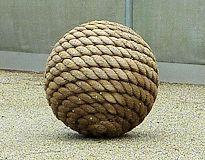There are about 8 spirals from the "North Pole" to the "equator" of the sphere.

The radius of the inner sphere is r = 7.08 cm, and the rope is 1.5 cm thick, so the radius of the (imaginary) sphere joining the center of each spiral arm is R = 7.08 + 0.5 × 1.5 = 7.83 cm.

In cross-section, it looks something like this: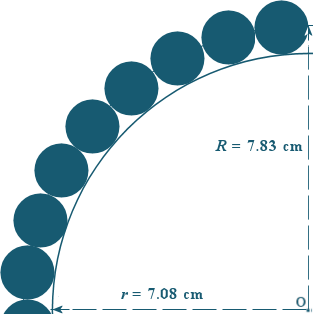The photo suggests the rope is actually flattened somewhat (not circular in cross-section as per my above image), but for the sake of this discussion, we'll assume the cross sections are circular.

Like all problems, there's more than one way to approach a solution. Let's look at 3 possible ways.

## Solution 1: Using calculus

We need to model the spiral using parametric coordinates. (See the introduction to this concept in the paraboloid problem.)

I started with the following coordinates, since they will give me equidistant spirals.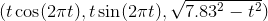(When we say "equidistant", we mean the spacing between each spiral arm when intersected by a "meridian" line will be equal.)

The x- and y- coordinates that I've chosen send a point around a spiral starting at x = 0, y = 0 when t = 0, and the z-coordinate is based on Pythagoras' Theorem, considering our sphere formed by the rope has radius 7.83 cm.

I'm just considering the top half of the spiral for now. In this case, the spiral starts at the "North Pole" (when t = 0).

From the top it looked good. The spirals are equally distant along each axis, and we have around 8 spirals as required: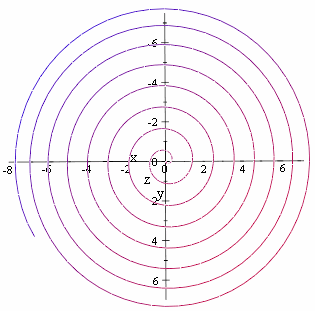However, when we look at the spiral from the side, there is a problem. The spirals get further apart as we get near the "equator".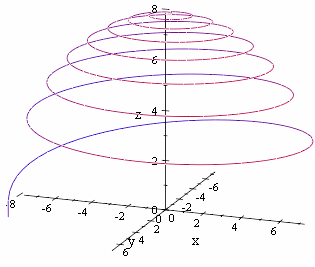The spiral length calculation would be reasonable for the first few spirals near the top, but would become increasingly inaccurate as t approaches 7.83 (the equator).

Let's try a different model.

This time I'm using the following parametric coordinates: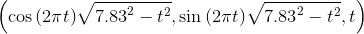Our graph starts from the "equator" this time. From the side it looks much better, since the spirals appear to be reasonably equally distant.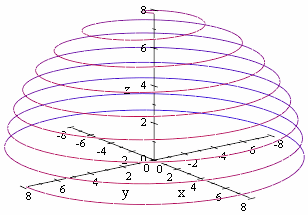However, let's now look near the top.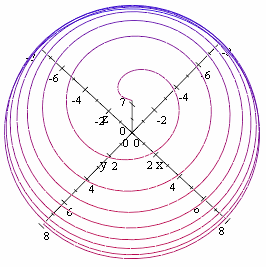Unfortunately, the spirals get further apart near the North Pole, so we have encountered a similar problem.

So, it was back to basics. I needed to modify the parametric equation for a sphere to suit the current situation.

### Parametric Coordinates for a Sphere

In general, a sphere is given by:

x = r cos(θ) cos(t)

y = r cos(θ) sin(t)

z = r cos(θ),

where 0 ≤ θ ≤ 2π and 0 ≤ t ≤π.

For our spiral, I tweaked the above and used:

x = 7.83 cos((t − π)/2) cos(16.4t)

y = 7.83 cos((t − π)/2) sin(16.4t)

z = 7.83 sin((t − π)/2),

where

0 ≤ t ≤ 2π.

This gives me just over 8 spirals in the top half, as required.

Here's the resulting spiral. I drew this one using GeoGebra, with maximum curve thickness.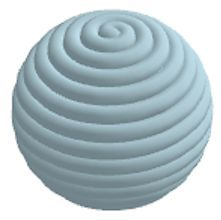I'm now ready to calculate the length of my spiral.

I will use the formula for the length of a curve in parametric coordinates: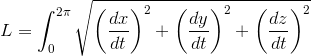A computer algebra system gives me the length as:

L = 514.9 cm = 5.15 m.

I must point out the situation in this problem is not particularly exact, influenced by how tight the rope is packed, how easy it is to reduce spaces near the top and bottom of the sphere due to the stiffness of the rope, and so on. However, in an ideal world, this is the length we could expect. It's quite close to Anne's "practical test" prediction.

## Solution 2: Non-calculus approach

Anne continued to play around with the problem while waiting for me to come up with something, and she proposed a very neat solution, much easier than the above. Here is Anne's approach:

The surface area of a sphere is given by:

S.A. = 4 π r2

In this case, the rope will cover an area of

S.A. = 4 π (7.83)2 = 770.4 cm2

Since the rope is 1.5 cm wide, the length of rope will be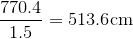This is quite close to my calculus-based answer, and it's certainly a neat way to do it!

## Solution 3: Estimate using equidistant circles

Another approach could be as follows. We could find the circumference of the circles that are equally spaced around the sphere (like "parallels of latitude").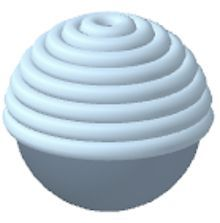To obtain equal spacing, I used the fact that the angle from the center between each circle would be π/16. Then the radius of the first one at the top would be 7.83 sin π/16, and its vertical distance from the center of the sphere would be 7.83 cos π/16.

A typical circle will have parametric equation (e.g. this is the 5th one from the top):

7.83sin(5π/16) cos(t), 7.83sin(5π/16) sin(t), 7.83 cos(5π/16)

It's now easy to find the circumference of these circles using C = 2πr. We add the circumferences to give:

L = (2π)(7.83 + 2[7.68 + 7.23 + 6.51 + 5.53 + 4.35 + 3.00 + 1.53]) = 541.0 cm

I only included the "equator" once.

This over-estimates the answer somewhat, but considering we wanted to know how much rope to buy, that's not a bad problem.

## Conclusion

The best solutions are usually the simplest ones!

### 9 Comments on “How to find the length of a spherical spiral”

1. cccccttttt says:

Well presented.

Will show students when we cover
parametric equations.

ct

2. Murray says:

3. Andrew says: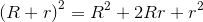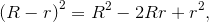instead of loose ends, maybe the rope could wind around inside the first spiral, to create a closed loop,

outside spiral + inside spiral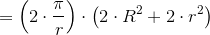4. Murray says:

Good idea, Andrew!

(I edited your answer a bit, since the math input system has certain keywords like "in" which is output as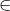.)

5. Andrew says:

Length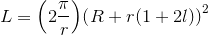Between the top and bottom of the sphere the number of Spiral stripes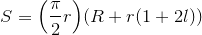first layer of spiral, l = 0, the next layer, l = 1, the first layer inside, l = -1.

6. Peter Farrell says:

Thank you for the equation of the helix. Worked perfectly for my purposes (drawing a helix in 3D). Since I used line segments to draw my helix, I suppose I could approximate the length by simply adding the lengths of the segments. The more segments the better the approximation.

7. Dhiraj Raj says:

can i use this equation in comsol for modelling hemispherical helix or spiral?

8. Murray says:

@Dhiraj: I can't see any reason why not.

9. Max says:

A shorthand estimate:

How about dividing the Area of the Sphere (4 Pi r 2) by the diameter of the rope?

I would guess adding the rope radius, to the spear radius in te area calculation would improve the estimate.

### Comment Preview

HTML: You can use simple tags like <b>, <a href="...">, etc.

To enter math, you can can either:

1. Use simple calculator-like input in the following format (surround your math in backticks, or qq on tablet or phone):
a^2 = sqrt(b^2 + c^2)
(See more on ASCIIMath syntax); or
2. Use simple LaTeX in the following format. Surround your math with $$ and $$.
$$\int g dx = \sqrt{\frac{a}{b}}$$
(This is standard simple LaTeX.)

NOTE: You can mix both types of math entry in your comment.